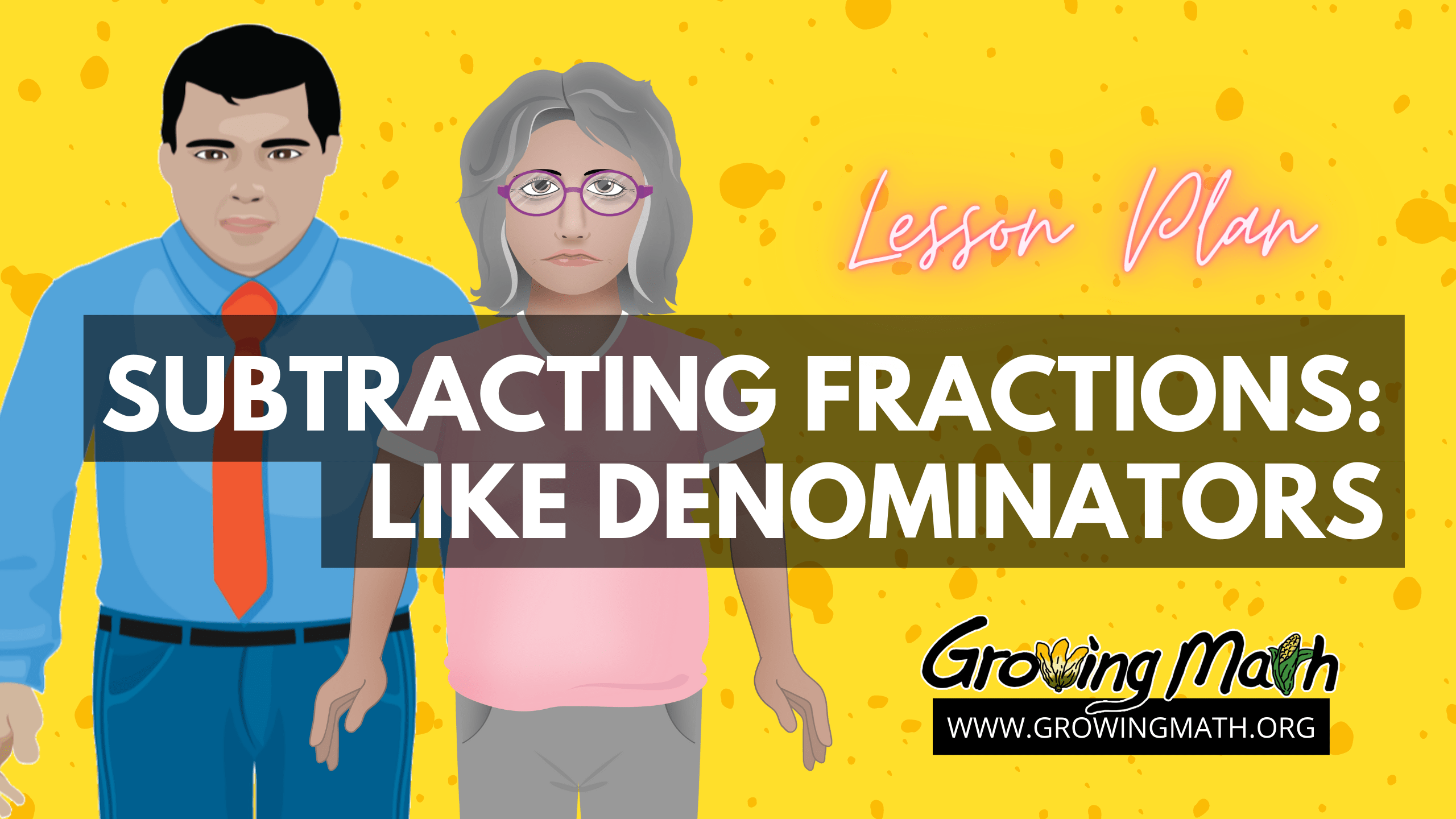# Subtracting Fractions: Like denominators

## 📖 Standards

CCSS.Math.Content.5.NF.A.2 Solve word problems involving addition and subtraction of fractions referring to the same whole, including cases of unlike denominators, e.g., by using visual fraction models or equations to represent the problem. Use benchmark fractions and number sense of fractions to estimate mentally and assess the reasonableness of answers. For example, recognize an incorrect result 2/5 + 1/2 = 3/7, by observing that 3/7 < 1/2.

## 📃 Summary

After this lesson, students will know how to solve multi–step word problems using addition and subtraction of fractions with like (common) denominators. After watching the video, students will login to “Aztech: The Story Begins” on a device with the website or application. Students will be faced with a fractions problem in Level 1 which uses a calendar to find the fraction of days students did homework. The game character points out that 16/31 may not be “all the time” but it is still more than half. Throughout the game, students will be presented with AzTech history.

## ⏰ Time required

30 -45 minutes, including individual assessment

## 📲 Technology required

The game in this lesson plan can be played on the web on any Chromebook, Mac or Windows computer with reliable Internet access. If students do not have high-speed Internet at home, the game can be pre-loaded on to iPads and played offline with no Internet required.

## 📚 Lesson Plan

### 1. Video: Adding Like Fractions

“Like fractions” are those with the same denominator. This is also called a common denominator. How do you add like fractions? This quick video from the game Fish Lake has simple examples of comparing fractions and fraction addition.

### 2. Presentation or video: When is a fraction the same as 1 ?

If the numerator and denominator are the same, then this fraction equals 1. N/N = 1 How can you apply your knowledge of fractions to help you figure out how far you’ve gone on your trip and how much further you have to go? Teachers can either have students watch the video or use this 27-slide presentation in both Google slides format and PowerPoint. Both include examples of fractions of 8/8 , 3/3 and 4/4 all equaling one. Examples include distance, money and a bowl of stew.

### 3. Game: Play AzTech – The Story Begins

Students will login to “Aztech: The Story Begins” on a device with the website or application. Students using Chromebook, Mac or Windows computers can play on the web here. Students using an iPad can download the app here. Throughout the game, students will be presented with Aztech history. Students will be faced with fraction and statistic examples, leading to similar problems that need to be solved.

Estimated time for this portion: 10 minutes.

## Assessment

### Individual Assessment

Use this template to have students create their own fraction equation.

It includes a calendar template and these instructions:

Use this template to show what you did most in the last month when you weren’t in school.

• Second, put a 1 in the calendar for the first day of this month and continue until all days of the month are filled.
• Third, make a picture or write what you did each day in each of the boxes.
• Fourth, write your own fraction equation like this:
• On 11/31 of the days, I played games on the computer.
• On  7/31 of the days I worked planting my garden
• On 13/31 of the days I was doing homework.

#### 11/31 + 7/31 + 13/31  =   31/31

Of course, if there are 28 or 30 days in the month, your denominator will be different.

### Group Assessment

Use the video below to solve the problem from Level 1 in AzTech: The Story Begins as a group. This video shows the problem from level 1 on finding the fraction of days Xitlali did homework and gives a hint on how to solve it. Ask the students why Xitlali said that 16/31 was more than half. How did she know? Introduce the concept of equivalent fractions.

State Standards

Minnesota State Standard 4.1.2.3 – Use fraction models to add and subtract fractions with like denominators in real-world and mathematical situations. Develop a rule for addition and subtraction of fractions with like denominators.